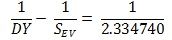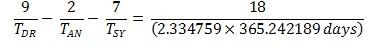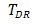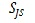Wednesday, November 25, 2015

Previously established facts about the lunar influence upon the Quasi-Biennial Oscillation (QBO).

Pukite  has identified four spectral peaks that contribute the greatest power to his QBO model.

These are:

2.370 years________54.6________________Draconic month annually aliased
2.528 years________25.3________________Mf' annually aliased
2.715 years________22.1________________Tropical month annually aliased
1.960 years________21.6________________half x (the anomalistic cycle annually aliased)

These have been previously identified by Vaughan  who has attributed their discovery to Piers Corbyn on or before the 29th November 2009.

[N.B. Mean Parameters used (J2000) in this analysis are:
Synodic month = 29.5305889 days
anomalistic month = 27.554550 days
Draconic month = 27.212221 days
Tropical month = 27.321582 days

Tropical year = 365.242189 days]

Vaughan, himself has shown that:

a) 1.960 tropical years  - half x (the anomalistic lunar monthly cycle annually aliased).

anomalistic month = 27.554550 days
nearest harmonic of tropical year:
(365.242189) / 13 = 28.095553 days

physical aliasing:
0.5 * (28.095553)*(27.554550) / (28.095553 – 27.554550) = 715.486162 days

(865.5210016) / 365.242189 = 1.958936 tropical years which rounds to 1.959 tropical years.

b) 2.37 tropical years - the Draconic lunar monthly cycle annually aliased

Draconic month = 27.212221 days
nearest harmonic of tropical year:
(365.242189) / 13 = 28.095553 days

physical aliasing:
(28.095553)*(27.212221) / (28.095553 – 27.212221) = 865.5210016 days

(865.5210016) / 365.242189 = 2.369718 tropical years which rounds to 2.37 tropical years.

[N.B. this almost exactly twice the nominal mean period for the Earth's Chandler wobble
of 432.8 days i.e. 2 x 432.8 days = 2.370 tropical years]

c) 2.528 tropical years [This is my little contribution]

This very close to the harmonic mean of 2.715 and 2.370 i.e.

2 (2.369718 x 2.715425) /(2.369718 + 2.715425) = 2.530930 tropical years
which rounds to 2.531 tropical years

d) 2.715 tropical years - the Synodic or the Tropical lunar monthly cycles annually aliased.

Synodic month = 29.5305889 days
nearest harmonic of tropical year:
(365.242189) / 12 = 30.43684908 days

physical aliasing:
(30.43684908)*(29.5305889) / (30.43684908 – 29.5305889) = 991.7881136 days
(991.78821136) / 365.242189 = 2.715426 tropical years
which rounds to 2.715 tropical years.

OR

Tropical month = 27.321582 days
nearest harmonic of tropical year:
(365.242189) / 13 = 28.095553 days

physical aliasing:
(28.095553)*(27.321582) / (28.095553 – 27.321582) = 991.7877480 days
(991.7877480) / 365.242189 = 2.715425 tropical years
which rounds to 2.715 tropical years.

CHECK:

As an added check, the above analysis indicates that if we take the beat period between the 2.370 tropical year aliased QBO Draconic period by the 2.715 tropical year aliased QBO Synodic period i.e.

2.715426 x 2.369718 / (2.715426 - 2.369718) = 18.61338 tropical years

we should get the same period as beat of the Draconic year (=346.62007589 days) with the tropical year i.e.

(365.242189 x 346.62007588) / (365.242189 - 346.62007589) = 6798.383967 days
__________________________________________________= 18.61336 tropical years.

References:

 http://contextearth.com/2015/11/07/more-refined-fit-of-qbo/#comment-174237

 https://tallbloke.wordpress.com/suggestions-14/comment-page-1/#comment-109035

 https://tallbloke.wordpress.com/suggestions-15/comment-page-1/#comment-109392

Thursday, November 12, 2015

A link between the Lunar tidal cycles and the planetary orbital periods of Venus, Earth, Jupiter and Saturn

SUMMARY OF RESULTS:The 2.334740 tropical year lunar tidal period is effectively just the synodic product of the lunar Draconic Year (DY) with the Synodic period of Venus and the Earth.There is a link between the synodic orbital periods of Venus/Earth and Jupiter/Saturn with the Lunar Nodal Cycle (LNC), Lunar Anomalistic Cycle (LAC), and the lunar Draconic Year (DY).

[N.B. all values above are in tropical years]

N.B. In addition, a connection was found between the synodic orbital periods of Venus/Earth and Jupiter/Saturn and the LAC when the time variables were expressed in sidereal years.

http://astroclimateconnection.blogspot.com.au/2015/05/the-six-year-re-alignment-period.html

START OF MAIN POST:

In an earlier post located at:

http://astroclimateconnection.blogspot.com.au/2015/11/two-new-connections-between-planetary.html

A. It was established that if you took the minimum period between the times of maximum change in the tidal stresses acting upon the Earth that are caused by changes in the direction of the lunar tides (i.e. 1.89803 tropical years = 2.0 Draconic years), and amplitude modulate this period by the minimum period between the times of maximum change in tidal stresses acting upon the Earth that are caused by changes in the strength of the lunar tides (i.e. 10.14686 tropical years = 9.0 Full Moon Cycles), you found that the 1.89803 year tidal forcing term is split into a positive and a negative side-lobe, such that:

Positive side-lobe
[10.14686 x 1.89803] / [10.14686 – 1.89803] = 2.3348 tropical yrs = 28.02 months    (1)

Negative side-lobe
[10.14686 x 1.89803] / [10.14686 + 1.89803] = 1.5989 tropical yrs                               (2)

where

10.146856 tropical years = 3706.059873 days = 9.0 Full Moon Cycles (FMC) and 1.0 FMC = 411.78443029 days = 1.127428 tropical years is the synodic beat period between the mean anomalistic month and the mean Synodic month.
1.89803 tropical years = 693.2401518 days = 2.0 Draconic Years and
1.0 Draconic Year = 346.62007588 days = 0.949014 tropical years is the synodic beat period between the between the mean Draconic month and the mean Synodic month.
Equation (1) can be rewritten as:(3)
and equation (2) can be rewritten as:(4)
where= the Draconic month = 27.212221 days= the anomalistic month = 27.554550 days= the Synodic month = 29.5305889 days

and 1.598939 tropical years = 583.999980 days  which differs from the Synodic period of Venus and the Earth by only 1.90 hours.

Adding equations (3) and (4) gives you:(5)

where= 1.598939 tropical years, which differs from the synodic period of Venus and the Earth by only 1.90 hours.

DY = 346.6190478 days = 0.9490115 tropical years,  which differs from the Draconic year by only 1.48 minutes.

Hence, the 2.334740 tropical year lunar tidal period is effectively just the synodic product of the lunar Draconic Year (DY) with the Synodic period of Venus and the Earth.

B.  It was established that:(6)

where= the Synodic period of Jupiter and Saturn = 19.8596 tropical years
LNC = Lunar Nodal Cycle = 18.6000 tropical years
LAC = Lunar anomalistic Cycle = 8.8505 tropical years

which, with the substitution of equation (5), can be rewritten as:(7)

Hence, equation (7) shows that there is a link between the synodic orbital periods of Venus/Earth and Jupiter/Saturn with the Lunar Nodal Cycle (LNC), Lunar Anomalistic Cycle (LAC), and the lunar Draconic Year (DY).

Friday, November 6, 2015

Two new connections between the Planetary and Lunar Cycles

updated: 09/11/2015 (see bottom of post)

1. The Connection Between the Lunar Tidal Cycles and the Synodic Period of Venus and the Earth.
The first direct connection between the planetary orbital periods and the lunar tidal cycles can be found in a previous blog post that is located at:
In this post it was found that:
If you take the minimum period between the times of maximum change in the tidal stresses acting upon the Earth that are caused by changes in the direction of the lunar tides (i.e. 1.89803 tropical years), and amplitude modulate this period by the minimum period between the times of maximum change in tidal stresses acting upon the Earth that are caused by changes in the strength of the lunar tides (i.e. 10.14686 tropical years), you find that the 1.89803 year tidal forcing term is split into a positive and a negative side-lobe, such that:
Positive side-lobe
[10.14686 x 1.89803] / [10.14686 – 1.89803] = 2.3348 tropical yrs = 28.02 months

Negative side-lobe
[10.14686 x 1.89803] / [10.14686 + 1.89803] = 1.5989 tropical yrs

N.B.
10.146856 tropical years = 3706.059873 days = 9.0 Full Moon Cycles (FMC) and
1.0 FMC = 411.78443029 days = 1.127428 tropical years.
1.89803 tropical years = 693.2401518 days = 2.0 Draconic Years and
1.0 Draconic Year = 346.62007588 days = 0.949014 tropical years.
The time period of the positive side-lobe is almost exactly the same as that of the Quasi-Biennial Oscillation (QBO). The QBO is a quasi-periodic oscillation in the equatorial stratospheric zonal winds that has a mean period of oscillation of approximately 28 months.
Even more remarkable is the time period of the negative side-lobe. It is almost exactly the same as that of the synodic period of the orbits of Venus and the Earth (i.e. 583.92063 days = 1.5987 tropical years), agreeing to within an error of only ~ 1.8 hours.

2. The Connection Between the Lunar Tidal Cycles and the Synodic Period of Jupiter and Saturn.
The second  direct connection between the planetary orbital periods and the lunar tidal cycles comes from a relationship that links the period of the QBO to the lunar and planetary cycles.

(8/19.8592) + (8/18.6000) + (4/8.8505) = 3/(2.3348)

where

19.8592 tropical yrs = the synodic period of Jupiter and Saturn.
18.6000 tropical yrs = time for the lunar line-of-nodes to precess around the Earth w.r.t. the stars.
8.8505 tropical yrs = time for the lunar line-of-apse to precess around the Earth w.r.t. the stars.
2.3348 tropical yrs = 28.02 months approximately equal to the average length of the QBO.

This can be rewritten as:

(8/19.8592) + (8/9.0697) = 3/(2.3348)

Where 9.0697 tropical years is half the harmonic mean of 17.7010 ( = 2 x 8.8505) tropical years and 18.6000 tropical years. This is close to the 9.1 tropical year spectral peak that is known as the the quasi-decadal oscillation.

Hence, we have an expression where the first term on the left is a bi-decadal oscillation, the second term on the left is a quasi-decadal oscillation and the denominator of the first term on the right is near to, but not precisely at, the nominal QBO oscillation period of 2.371 tropical years.

Remarkably, however, the  denominator of the first term on the right-hand side is exactly that of the positive side-lobe produced by the amplitude modulation in part 1.

Hnece, we have established two new connections between the synodic periods of Earth/Venus and Jupiter/Saturn that directly link into the variations in the stresses placed upon the Earth's atmosphere and oceans by the luni-solar tidal cycles

UPDATE: 09/11/15

A third connection between the orbital period of Jupiter and the lunar Draconic year.

In my 2008 paper (that was eventually published in 2010) i.e.

Wilson, I.R.G., 2011, Are Changes in the Earth’s Rotation
Rate Externally Driven and Do They Affect Climate?
The General Science Journal, Dec 2011, 3811.

http://gsjournal.net/Science-Journals/Essays/View/3811

I showed that:

5.0 Draconic years = 0.4 Jupiter orbits = 4 x 433.275 days = 4 x Chandler wobble = 2 x QBO

(with 1 Jupiter orbit = 4332.75 days = 11.8627 tropical years)

This is equivalent to:

50 Draconic years = 4 x 11.8627 topical years = 4 x orbital period of Jupiter.

Thanks oldbrew for reminding every one of this additional connection between the Lunar Draconic cycle and orbit of Jupiter that I made back in 2008.